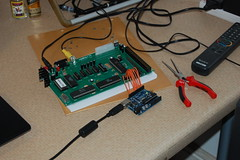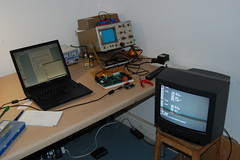# Archive for the ‘Arduino’ Category

## Projects: ASCII keyboard emulator for Apple I Replica

Posted by llemarie on February 20, 2010Last week I built an Apple I Replica from a Briel Computers kit. While I loved the completed machine, I suffered a bit from the fact that backspace doesn’t work (you have to type the assembly *exactly* correct), and of course it loses the RAM contents when shut down.

I wanted to make an interface to the PC so I could use a modern editor and simply copy-paste to the Apple I. As a quick and dirty solution to the problem, I used an Arduino, wired it to the ASCII keyboard port of the Apple I and wrote a small sketch that listens on the serial port and sets the data pins accordingly.

Photos on Flickr.

Here’s the Arduino sketch, real simple:

```/*
ASCII keyboard
Lionel Lemarie
2010-02

Listens on the serial port for characters from a PC.
Outputs the codes like an ASCII keyboard.
Compatible with Apple I Replica.
*/

// The order of the pins is chosen for minimal wire crossing
// when connected to an ASCII keyboard socket.
int ASCII0 = 7;
int ASCII1 = 8;
int ASCII2 = 3;
int ASCII3 = 5;
int ASCII4 = 4;
int ASCII5 = 6;
int ASCII6 = 2;
int STROBE = 10;
int NRESET = 9; //reset active low

// LED will blink when a character is emitted
int LED = 13;
unsigned long previousMillis = 0;
unsigned long interval = 200;

void setup()
{
analogReference(EXTERNAL); // Is this needed?

// Initialize the digital pins as output
pinMode(ASCII0, OUTPUT);
pinMode(ASCII1, OUTPUT);
pinMode(ASCII2, OUTPUT);
pinMode(ASCII3, OUTPUT);
pinMode(ASCII4, OUTPUT);
pinMode(ASCII5, OUTPUT);
pinMode(ASCII6, OUTPUT);
pinMode(STROBE, OUTPUT);
pinMode(NRESET, OUTPUT);

pinMode(LED, OUTPUT);

digitalWrite(STROBE, LOW);   // set the STROBE pin to inactive
digitalWrite(NRESET, HIGH);  // set the RESET  pin to inactive

Serial.begin(9600);
}

void loop()
{
unsigned long currentMillis = millis();

if (Serial.available() > 0)
{
iInput &= 127;

if (iInput)
{
if (iInput>='a' && iInput<='z')
iInput = iInput - 'a' + 'A';

int D6 = LOW;
int D5 = LOW;
int D4 = LOW;
int D3 = LOW;
int D2 = LOW;
int D1 = LOW;
int D0 = LOW;

if ( iInput & 64 ) D6 = HIGH;
if ( iInput & 32 ) D5 = HIGH;
if ( iInput & 16 ) D4 = HIGH;
if ( iInput &  8 ) D3 = HIGH;
if ( iInput &  4 ) D2 = HIGH;
if ( iInput &  2 ) D1 = HIGH;
if ( iInput &  1 ) D0 = HIGH;

digitalWrite(LED, HIGH);
previousMillis = currentMillis;

// Output an A (100 0001)
digitalWrite(ASCII6, D6);
digitalWrite(ASCII5, D5);
digitalWrite(ASCII4, D4);
digitalWrite(ASCII3, D3);
digitalWrite(ASCII2, D2);
digitalWrite(ASCII1, D1);
digitalWrite(ASCII0, D0);
digitalWrite(STROBE, HIGH);
delay(40);  // strobe for 40ms
digitalWrite(STROBE, LOW);
}
}

if ( (previousMillis>0) && (currentMillis-previousMillis>interval) )
{
digitalWrite(LED, LOW);
previousMillis = 0;
}
}
```